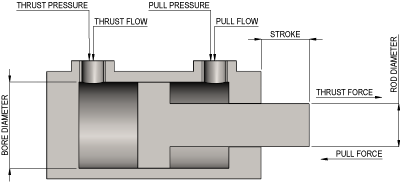## HYDRAULIC KNOWN CYLINDER CALCULATOR

If the dimensions of a hydraulic cylinder are known, then various volumetric calculations can be carried out, such as Full bore area and volumes.

If the hydraulic system pressure is also known then the Thrust Force and Pull Force can also be determined.

If the required cylinder speed is known then the Power required and Oil Flow rates can also be calculated.

To use this calculator enter your six known values and select units as required.

If only one cylinder is to be calculated a one must be entered.